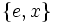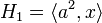Klein four-subgroups of dihedral group:D8

(diff) ← Older revision | Latest revision (diff) | Newer revision → (diff)
This article is about a particular subgroup in a group, up to equivalence of subgroups (i.e., an isomorphism of groups that induces the corresponding isomorphism of subgroups). The subgroup is (up to isomorphism) Klein four-group and the group is (up to isomorphism) dihedral group:D8 (see subgroup structure of dihedral group:D8).
The subgroup is a normal subgroup and the quotient group is isomorphic to cyclic group:Z2.
VIEW: Group-subgroup pairs with the same subgroup part | Group-subgroup pairs with the same group part| Group-subgroup pairs with the same quotient part | All pages on particular subgroups in groups

This article discusses the dihedral group of order eight (see details on the subgroup structure) and the two Klein four-subgroups of this group. We call the dihedral group$G$, and use the following presentation:$\! G = \langle a,x \mid a^4 = x^2 = e, xax = a^{-1} \rangle$

and we set:$\! H_1 = \langle a^2,x \rangle, \qquad H_2 = \langle a^2,ax \rangle$.

As full lists:$\! H_1 = \{ e, x, a^2, a^2x \}, \qquad H_2 = \{ e, ax, a^2, a^3x \}$

Cosets

Both subgroups have index two and are hence normal subgroups. Each of them has two cosets: the subgroup itself and the rest of the group.

The cosets of$H_1$ are:$\! H_1 = \{ e, x, a^2, a^2x \}, G \setminus H_1 = \{ ax, a^3x, a, a^3 \}$

The cosets of$H_2$ are:$\! H_2 = \{ e, ax, a^2, a^3x \}, G \setminus H_1 = \{ x, a^2x, a, a^3 \}$

Complements

COMPLEMENTS TO NORMAL SUBGROUP: TERMS/FACTS TO CHECK AGAINST:
TERMS: permutable complements | permutably complemented subgroup | lattice-complemented subgroup | complemented normal subgroup (normal subgroup that has permutable complement, equivalently, that has lattice complement) | retract (subgroup having a normal complement)
FACTS: complement to normal subgroup is isomorphic to quotient | complements to abelian normal subgroup are automorphic | complements to normal subgroup need not be automorphic | Schur-Zassenhaus theorem (two parts: normal Hall implies permutably complemented and Hall retract implies order-conjugate)$H_1$ has two permutable complements:$\{ e,ax \}$ and$\{ e, a^3x \}$, both of which are conjugate subgroups and subgroups of$H_2$.$H_2$ has two permutable complements:$\{ e, x \}$ and$\{ e, a^2x \}$, both of which are conjugate subgroups and are subgroups of$H_1$.

The four subgroups of order two arising as complements in this way are all automorphic subgroups. For information on these, see non-normal subgroups of dihedral group:D8.

Properties related to complementation

For convenience, we refer to$H_1$ below, though$H_2$ behaves the same way.

Property Meaning Satisfied? Explanation Comment
complemented normal subgroup normal subgroup with permutable complement Yes see above
permutably complemented subgroup subgroup with permutable complement Yes (via complemented normal)
lattice-complemented subgroup subgroup with lattice complement Yes (via permutably complemented)
retract has a normal complement No
direct factor normal subgroup with normal complement No

Arithmetic functions

Function Value Explanation
order of whole group 8
order of subgroup 4
index 2
size of conjugacy class 1
number of conjugacy classes in automorphism class 2

Effect of subgroup operators

In the table below, we provide values specific to$H_1$.

Function Value as subgroup (descriptive) Value as subgroup (link) Value as group
normalizer whole group$\langle a,x \rangle$ dihedral group:D8
centralizer the subgroup itself current page Klein four-group
normal core the subgroup itself current page Klein four-group
normal closure the subgroup itself current page Klein four-group
characteristic core$\langle a^2 \rangle$ center of dihedral group:D8 cyclic group:Z2
characteristic closure whole group dihedral group:D8
commutator with whole group$\langle a^2 \rangle$ center of dihedral group:D8 cyclic group:Z2

Automorphism class-defining functions

Below are some functions that start with the whole group as a black box group and give the unique automorphism class comprising the two Klein four-subgroups. Note that these functions are not guaranteed to always give a single automorphism class of subgroups for a general group.

Function Where does it make sense in general? Always gives unique automorphism class? Why it gives these subgroups?
elementary abelian subgroup of maximum order typically, context of group of prime power order No By inspection of subgroup structure
abelian subgroup of maximum rank typically, context of group of prime power order No By inspection of subgroup structure
non-characteristic normal subgroup any group No By inspection of subgroup structure

Related subgroups

Intermediate subgroups

There are no properly intermediate subgroups because the subgroup is a maximal subgroup.

Smaller subgroups

Below we discuss subgroups inside$H_1$.

Value of smaller subgroup (descriptive) Isomorphism class of smaller subgroup Smaller subgroup in subgroup Smaller subgroup in whole group$\langle a^2 \rangle$ cyclic group:Z2 Z2 in V4 center of dihedral group:D8$\langle x \rangle$ cyclic group:Z2 Z2 in V4 non-normal subgroups of dihedral group:D8$\langle a^2x \rangle$ cyclic group:Z2 Z2 in V4 non-normal subgroups of dihedral group:D8

Images under quotient maps

The discussion below is for$H_1 = \langle a^2, x \rangle$.

Kernel of quotient map (descriptive) Kernel of quotient map (link) Image of whole group as group Image of subgroup as group Embedding (link)$\langle a \rangle$ cyclic maximal subgroup of dihedral group:D8 cyclic group:Z2 cyclic group:Z2 Z2 in itself$\langle a^2, ax \rangle$ Klein four-subgroups of dihedral group:D8 cyclic group:Z2 cyclic group:Z2 Z2 in itself$\langle a^2 \rangle$ center of dihedral group:D8 Klein four-group cyclic group:Z2 Z2 in V4

Subgroup properties

Invariance under automorphisms and endomorphisms

Suppose$c_a$ and$c_x$ denote conjugation by$a$ and$x$ respectively. Let$\sigma$ denote the automorphism that sends$a$ to$a^3$ and$x$ to$ax$. Then,$\langle c_a, c_x\rangle$ is the inner automorphism group and$\langle c_a, c_x, \sigma \rangle$ is the automorphism group. It turns out that$\sigma(H_1) = H_2$ and$\sigma(H_2) = H_1$, while$c_a$ and$c_x$ preserve both$H_1$ and$H_2$. Note that the automorphism group is isomorphic to dihedral group:D8 and the inner automorphism group to the Klein four-group.

Note that since$\sigma$ sends$H_1$ to$H_2$, they both satisfy the same subgroup properties. For convenience, we use notation and symbols for$H_1$.

Property Meaning Satisfied? Explanation Comment
normal subgroup invariant under inner automorphisms Yes subgroup of index two is normal
coprime automorphism-invariant subgroup invariant under automorphisms of coprime order to group Yes there are no nontrivial automorphisms of coprime order
coprime automorphism-invariant normal subgroup normal and coprime automorphism-invariant Yes
characteristic subgroup invariant under all automorphisms No$\sigma(H_1) = H_2 \ne H_1$
cofactorial automorphism-invariant subgroup invariant under all automorphisms whose order has prime factors only among those of the group No$\sigma$ has order two, and$\sigma(H_1) \ne H_1$
fully invariant subgroup invariant under all endomorphisms No (via characteristic)

Property Meaning Satisfied? Explanation Comment
order-normal subgroup every subgroup of the same order is normal Yes maximal subgroup of group of prime power order is normal
isomorph-normal subgroup every isomorphic subgroup is normal Yes
isomorph-automorphic subgroup every isomorphic subgroup is automorphic Yes
order-isomorphic subgroup isomorphic to all subgroups of the same order No Cyclic subgroup$\langle a \rangle$ has same order, not isomorphic

Properties related to embeddings in bigger groups

Property Meaning Satisfied? Explanation Comment
isomorph-normal subgroup every isomorphic subgroup is normal Yes index two implies normal
coprime automorphism-invariant subgroup invariant under all automorphisms of coprime order Yes no non-identity automorphisms of coprime order.
fusion system-relatively weakly closed subgroup weakly closed subgroup for any fusion system on the whole group Yes isomorph-normal coprime automorphism-invariant implies fusion system-relatively weakly closed
Sylow-relatively weakly closed subgroup weakly closed subgroup whenever we embed dihedral group:D8 as a Sylow subgroup in a bigger group Yes isomorph-normal coprime automorphism-invariant implies Sylow-relatively weakly closed
finite-pi-potentially fully invariant subgroup there is a finite 2-group containing$G$ in which$H_1$ is fully invariant Yes homocyclic normal implies finite-pi-potentially fully invariant in finite
finite-pi-potentially characteristic subgroup there is a finite 2-group containing$G$ in which$H_1$ is characteristic Yes (via finite-pi-potentially fully invariant)
potentially fully invariant subgroup there is a group containing$G$ in which$H_1$ is fully invariant Yes (via finite-pi-potentially fully invariant)

Properties related to conjugacy and splitting and transitivity of normality

Property Meaning Satisfied? Explanation
central factor product with centralizer is whole group No The subgroup is a proper self-centralizing subgroup.
conjugacy-closed subgroup any two elements of the subgroup conjugate in the whole group are conjugate in the subgroup No The elements$x$ and$a^2x$ are conjugate in the whole group and not in the subgroup, which is abelian.
transitively normal subgroup every normal subgroup of the subgroup is normal in the whole group No The subgroup$\{ e, x \}$ (see non-normal subgroups of dihedral group:D8) inside$H_1$ is normal in$H_1$, not in$G$. See also normality is not transitive -- this is the smallest example.

Generic maximality notions$H_1$ and$H_2$ are both maximal subgroups of the group of prime power order$G$. Thus, they satisfy all these properties: maximal normal subgroup, maximal subgroup, subgroup of index two, order-normal subgroup, isomorph-normal subgroup, maximal subgroup of finite nilpotent group.

As already discussed, they are both also coprime automorphism-invariant, hence they are isomorph-normal coprime automorphism-invariant subgroup of group of prime power order. In particular, they are both fusion system-relatively weakly closed subgroups and thus Sylow-relatively weakly closed subgroups.

Maximality notions related to abelianness

Abelian subgroups of maximum order$H_1$ and$H_2$ are both abelian subgroups of maximum order. There is one more abelian subgroup of maximum order, namely the cyclic subgroup$\langle a \rangle$.

Elementary abelian subgroups of maximum order$H_1$ and$H_2$ are both elementary abelian subgroups of maximum order in$G$. There are no others.

Abelian subgroups of maximum rank$H_1$ and$H_2$ are both abelian subgroups of maximum rank in$G$. There are no others.

GAP implementation

Finding these subgroups inside a black-box dihedral group

Suppose$G$ is a group that we know is isomorphic to dihedral group:D8. We can create a two-element list of the Klein four-subgroups of$G$ using the command:

H := Filtered(NormalSubgroups(G), x -> IdGroup(x) = [4,2]);

We can then set:

H1 := H;
H2 := H;

Constructing the dihedral group and the two subgroups

This can be achieved by the sequence of commands:

G := DihedralGroup(8);
H := Filtered(NormalSubgroups(G), x -> IdGroup(x) = [4,2]);
H1 := H;
H2 := H;